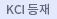#다국어 입력

http://chineseinput.net/에서 pinyin(병음)방식으로 중국어를 변환할 수 있습니다.

변환된 중국어를 복사하여 사용하시면 됩니다.

예시)
• 中文 을 입력하시려면 zhongwen을 입력하시고 space를누르시면됩니다.
• 北京 을 입력하시려면 beijing을 입력하시고 space를 누르시면 됩니다.
닫기

검색결과 좁혀 보기

• 좁혀본 항목 보기순서

• 원문유무
• 음성지원유무
• 원문제공처
• 등재정보
• 학술지명
• 주제분류
• 발행연도
• 작성언어
• 저자

오늘 본 자료

• 오늘 본 자료가 없습니다.
더보기
• 무료
• 기관 내 무료
• 유료
••Generalized difference sequence spaces

In the present paper, we will bring out the new techniques of combining the generalized B- dierence sequence spaces with binomial mappings and the Δ operator. Moreover, the basic properties of these spaces will be taken care of.

•Complete left ideals in ternary semigroups

In this paper we introduce the notions of complete leftideals in ternarysemigroups. We also define direct product and left unit of a ternarysemigroups. And obtain some results from these ideals. We obtain the relation between the complete leftideals and set of all (0, 1) regular elements of a ternarysemigroups.

•A study of steady laminar boundary layer flow and heat transfer along an infinite, porous, hot, vertical continuous moving plate in the presence of heat source and constant free stream

An analysis is made for the study of two- dimensional laminar boundary layer flow of a viscous, incompressible fluid, along an infinite, porous, hot, vertical continuous moving plate. The governing partial differential equations are non-dimensionalized and are solved using Natural Transform Technique. The expressions for velocity field, temperature field, rate of heat transfer and skin-friction have been obtained. The influence of various physical parameters, such as Eckert number Ec, Prandtl number Pr, Grashoff number Gr, plate velocity α and heat source/sink parameters is extensively discussed with the help of graphs to show the physical aspects of the problem. It is found that these parameters significantly affect the flow and heat transfer.

•Distance majorization integrity of graphs

The concept of distance majorization integrity is introduced as a new measure of the stability of a graph G and it is dened as DMI(G) = min{|S| + m(G - S)}, where S is a distance majorization set and m(G - S) is the order of a maximum component of G - S. The distance majorization integrity of some graphs is obtained. The relations between distance majorization integrity and other parameters are determined. Also a distance majorization integrity of corona of some graphs are computed

•DEGENERATE COMPLETE BELL POLYNOMIALS AND NUMBERS

Recently, several authors have studied complete Bell polyno- mials and numbers. In this paper, we consider degenerate complete Bell polynomials and numbers and we give some explicit formulas for these numbers and polynomials related to degenerate Stirling numbers.

•LIST OF DEGENERATE FINITE-DIMENSIONAL QUASIREPRESENTATIONS OF GROUPS

We apply well-known results concerning finite-dimensional quasirepresentations of groups to make a list of finite-dimensional quasi-representations some of whose characterizing subspaces vanish.

•NOTE ON MULTIPLE q-ZETA FUNCTIONS

In this paper we consider the analytic continuation of the multiple Euler q-zeta function in the complex number field as follows: ζEr,q(s,x)=rq∞∑m1,…,mr=0(-1)m1+…+mr/[x+m1+…+mr]sq, where q ∈ C with |q| < 1, ℜ(x) > 0, and r ∈ N. Thus, we investigate their behavior near the poles and give the corresponding functional equations.

•FOURIER SERIES OF FUNCTIONS RELATED TO BERNOULLI POLYNOMIALS

We study the Fourier series of functions related to Bernoulli polynomials. As consequences, several new identities for the Bernoulli functions and numbers are derived.

•On partially degenerate Bell numbers and polynomials

Recently, several authors have studied Bell numbers and poly- nomials, also called Tochard polynomials or exponential polynomials, by using and without using umbral calculus. In this paper, as a degenerate ver- sion of ordinary Bell numbers and polynomials, we introduce the partially degenerate Bell numbers and polynomials and give some new identities for those numbers and polynomials related with Stirling numbers of the rst and second kind.